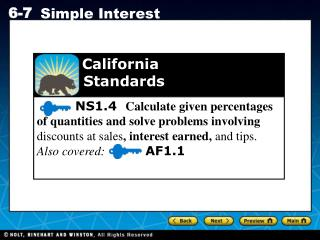DownloadDownload PresentationCalifornia Standards

# California Standards

Télécharger la présentation## California Standards

- - - - - - - - - - - - - - - - - - - - - - - - - - - E N D - - - - - - - - - - - - - - - - - - - - - - - - - - -
##### Presentation Transcript

1. California Standards NS1.4 Calculate given percentages of quantities and solve problems involving discounts at sales,interest earned, and tips. Also covered:AF1.1

2. Vocabulary interest simple interest principal

3. When you keep money in a savings account, your money earns interest. Interest is an amount that is collected or paid for the use of money. For example, the bank pays you interest to use your money to conduct its business. Likewise, when you borrow money from the bank, the bank collects interest on its loan to you.

4. One type of interest, called simple interest, is money paid only on the principal. The principal is the amount of money deposited or borrowed. To solve problems involving simple interest, you can use the following formula. Simple interest is money paid only on the principal Rate of interest is the percent charged or earned I= P · r · t Time that the money is borrowed or invested (in years) Principal is the amount borrowed or invested Notice that the interest rate is expressed as a decimal and the time is expressed in years.

5. Additional Example 1: Using the Simple Interest Formula Find the missing value. A. I = , P =\$575, r =8%, t = 3 years I =P · r · t I =575 · 0.08 · 3 Substitute. Use 0.08 for 8%. I = \$138 Multiply. The simple interest is \$138.00.

6. 10,200r 10,200 204 10,200 = Additional Example 1: Using the Simple Interest Formula Find the missing value. B. I = \$204, P = \$1,700, r = , t = 6 years I =P · r · t 204= 1,700 · r · 6 Substitute. 204 = 10,200r Multiply. Divide each side by 10,200. 0.02 = r The interest rate is 2%.

7. Check It Out! Example 1 Find the missing value. A. I = , P =\$525, r =7%, t = 2 years I =P · r · t I =525 · 0.07 · 2 Substitute. Use 0.07 for 7%. I = \$73.50 Multiply. The simple interest is \$73.50.

8. 6,000r 6,000 600 6000 = Check It Out! Example 1 Find the missing value. B. I = \$600, P = \$2,000, r = , t = 3 years I =P · r · t 600= 2,000 · r · 3 Substitute. 600 = 6,000r Multiply. Divide each side by 6,000. 0.1 = r The interest rate is 10%.

9. 1 Understand the Problem Additional Example 2: Problem Solving Application Avery deposits \$6,000 in an account that earns 4% simple interest. How long will it take for his account balance to reach \$6,800? Rewrite the question as a statement: • Find the number of years it will take for Avery’s account to reach \$6,800. List the important information: •The principal is \$6,000. • The interest rate is 4%. • His account balance will be \$6,800.

10. Make a Plan 2 Additional Example 2 Continued Avery’s account balance A includes the principal plus the interest: A = P + I. Once you solve for I, you can use I = P · r · t to find the time.

11. 3 Solve. –6,000 – 6,000 800 240 240t 240 = Additional Example 2 Continued A = P + I Substitute. 6,800 = 6,000 + I Subtract from each side. 800 = I I = P · r · t 800 = 6,000 · 0.04 · t Substitute. Use 0.04 for 4%. Multiply. 800 = 240t Divide each side by 240. 3.33 t Round to the nearest hundredth. 1 3 It will take 3 years.

12. 4 Additional Example 2 Continued Look Back 1 3 After exactly 3 years, Avery’s money will have earned \$800 in simple interest and his account balance will be \$6,800. 1 3 = 800 I = 6,000 · 0.04 · 3 1 3 An answer of 3 years for the account to reach \$6,800 makes sense.

13. 1 Understand the Problem Check It Out! Example 2 Linda deposits \$10,000 in an account that earns 8% simple interest. How long will it take for the total amount in her account to reach \$12,000? Rewrite the question as a statement: • Find the number of years it will take for Linda’s account to reach \$12,000. List the important information: •The principal is \$10,000. • The interest rate is 8%. • Her account balance will be \$12,000.

14. Make a Plan 2 Check It Out! Example 2 Continued Linda’s account balance A includes the principal plus the interest: A = P + I. Once you solve for I, you can use I = P · r · t to find the time.

15. 3 Solve –10,000 –10,000 2,000 800 800t 800 = Check It Out! Example 2 Continued A = P + I Substitute. 12,000 = 10,000 + I Subtract 10,000 from each side. 2,000 = I I = P · r · t 2,000 = 10,000 · 0.08 · t Substitute. Use 0.08 for 8%. 2,000 = 800t Multiply. Divide each side by 800. 2.5 = t 1 2 It will take 2 years.

16. 4 Check It Out! Example 2 Continued Look Back 1 2 After exactly 2 years, Linda’s money will have earned \$2,000 in simple interest and her account balance will be \$12,000. 1 2 = 12,000 I = 10,000 · 0.08 · 2 1 2 An answer of 2 years for the account to reach \$12,000 makes sense.